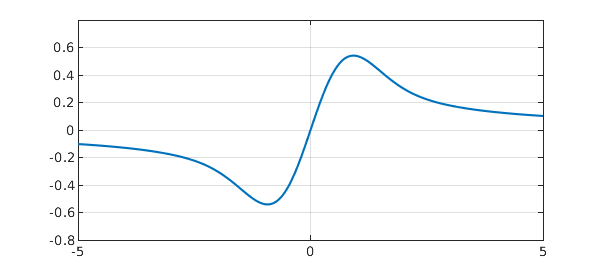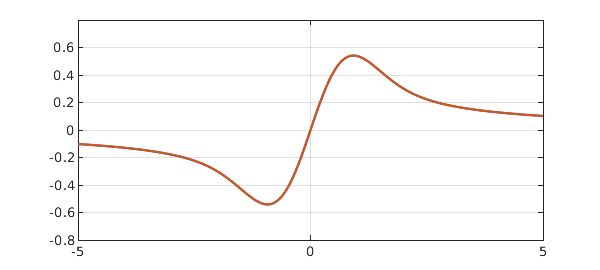Here is a simple linear ODE boundary value problem:

$${dF\over dx} + 2xF = 1, \qquad F(0) = 0.$$

Chebfun can crack this problem in a few lines. Instead of a boundary condition, we will specify an interior point condition.

function DawsonIntegral
LW = 'linewidth'; lw = 2;
tic
W = 5; H = 0.8;
L = chebop(-W,W);
L.op = @(x,f) diff(f,1) + 2*x.*f;   % ODE
L.bc = @(x,f) f(0);                 % interior point condition
f = L\1;
toc
plot(f,LW,lw), axis([-W W -H H]), hold on, grid on;
Elapsed time is 2.369714 seconds.The problem can be solved analytically:

$$F(x) = e^{-x^2} \int_0^x e^{t^2} dt.$$

Users with access to the MATLAB Symbolic Toolbox could also solve it with the following code:

y = sym('y');
f(y) = sym('f(y)');
f = dsolve(diff(f) + 2*y*f == 1, f(0) == 0);
pretty(f);

Equation (2) is known as Dawson's integral or Dawson's function, featuring a dipole structure about the origin. With the Symbolic Toolbox, you could plot the exact solution like this:

fexact = chebfun(@(x) mfun('dawson', x), [-W W]);
plot(fexact, '-.r'), hold off

On my machine, running the last few lines takes about 0.13 seconds.

It's tempting to evaluate Dawson's integral directly using Chebfun.

tic
x = chebfun('x',[0,W]);
fr = exp(-x.^2).*cumsum(exp(x.^2));  % right of x=0
fl = newDomain(-flipud(fr),[-W 0]);  % left of x=0
f = join(fl,fr);                     % must be an easier way to do this!
f = merge(f)
plot(f,LW,lw), grid on
f =
chebfun column (1 smooth piece)
interval       length   endpoint values
[      -5,       5]       38      -0.1      0.1
Epslevel = 9.665300e-07.  Vscale = 5.396731e-01.How big is the discrepancy between $F$ and $f$? You can find out by running these three lines:

semilogy(abs(f-fexact));
title('error when evaluate F directly');
grid on, hold off

If you do, you'll find that the accuracy is only about 5 digits. It's not difficult to understand the low accuracy if we notice that Dawson's integral as shown in Equation (2) is a product of type $0 \cdot \infty$ as $x$ diverges away from the origin. A standard way to compute Dawson's integral is given in Numerical Recipes , where the integral is evaluated using its Maclaurin series [2,3] near the origin and Rybicki's exponentially accurate approximation  otherwise. It's very likely that MATLAB's built-in routine adopts this algorithm.

An elegant way to evaluate Dawson's integral as well as several others in the complex error function family has been proposed by Weideman . Here we borrow Weideman's eight-line MATLAB code (very slightly modified) to calculate the integral.

N = 36;
tic
f = chebfun(@(x) real(sqrt(pi)*(cef(x,N)-exp(-x.^2))/2i), [-W W]);
toc
Elapsed time is 0.051382 seconds.


If you have the Symbolic Toolbox...

semilogy(abs(f-fexact)), grid on
function w = cef(z,N)      % Weideman's complex error function routine
M = 2*N;  M2 = 2*M;  k = (-M+1:1:M-1)';      % M2 = no. of sampling points.
L = sqrt(N/sqrt(2));                         % Optimal choice of L.
theta = k*pi/M; t = L*tan(theta/2);          % Define variables theta and t.
f = exp(-t.^2).*(L^2+t.^2); f = [0; f];      % Function to be transformed.
a = real(fft(fftshift(f)))/M2;               % Coefficients of transform.
a = flipud(a(2:N+1));                        % Reorder coefficients.
Z = (L+1i*z)./(L-1i*z); p = polyval(a,Z);    % Polynomial evaluation.
w = 2*p./(L-1i*z).^2+(1/sqrt(pi))./(L-1i*z); % Evaluate w(z).
end

Weideman's algorithm takes advantage of a slick rational expansion which approximates the Faddeeva function

$$w(x) = e^{-x^2}+\frac{2i}{\sqrt{\pi}}e^{-x^2}\int_0^x e^{t^2} dt$$

uniformly accurately in the complex plane with only a small number of terms (denoted by $N$ in the code above). With $N = 36$, Dawson's integral is computed accurately within roundoff and it's done roughly ten times faster than the MATLAB Symbolic Toolbox built-in function. Amazing, isn't it? Should we suggest to MathWorks that they rewrite their Dawson's function after an 18-year delay?

end
1. W. H. Press, S. A. Teukolsky, W. T. Vetterling, and B. P. Flannery, Numerical Recipes. The Art of Scientific Computing. Third edition. Cambridge University Press, Cambridge, 2007.

2. G. B. Rybicki, Dawson's integral and the sampling theorem. Computers in Physics, vol. 3 (1989), no. 2, pp. 85-87.

3. http://en.wikipedia.org/wiki/Dawson_function

4. W. J. Cody, K. A. Pociorek, and H. C. Thatcher, Chebyshev approximations for Dawson's integral. Mathematics of Computation, vol. 24 (1970), pp. 171-178.

5. J. A. C. Weideman, Computation of the complex error function. SIAM Journal on Numerical Analysis, 31 (1994), no. 5, 1497-1518.